Next: Aerodynamic Networks Up: Types of analysis Previous: Stationary groundwater flow   Contents

### Diffusion mass transfer in a stationary medium

The governing equations for diffusion mass transfer are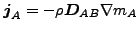(335)

and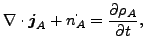(336)

where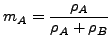(337)

and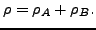(338)

In these equations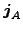is the mass flux of species A,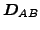is the mass diffusivity,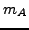is the mass fraction of species A and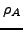is the density of species A. Furthermore,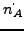is the rate of increase of the mass of species A per unit volume of the mixture. Another way of formulating this is: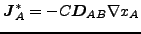(339)

and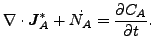(340)

where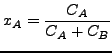(341)

and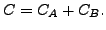(342)

Here,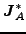is the molar flux of species A,is the mass diffusivity,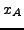is the mole fraction of species A and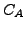is the molar concentration of species A. Furthermore,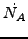is the rate of increase of the molar concentration of species A.

The resulting equation now reads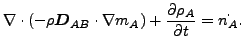(343)

or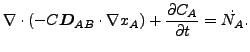(344)

If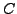andare constant, these equations reduce to: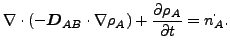(345)

or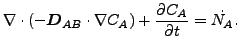(346)

Accordingly, by comparison with the heat equation, the correspondence in Table  (16) arises.

 heat mass diffusion T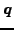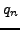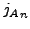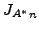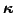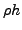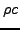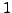Next: Aerodynamic Networks Up: Types of analysis Previous: Stationary groundwater flow   Contents
guido dhondt 2018-12-15